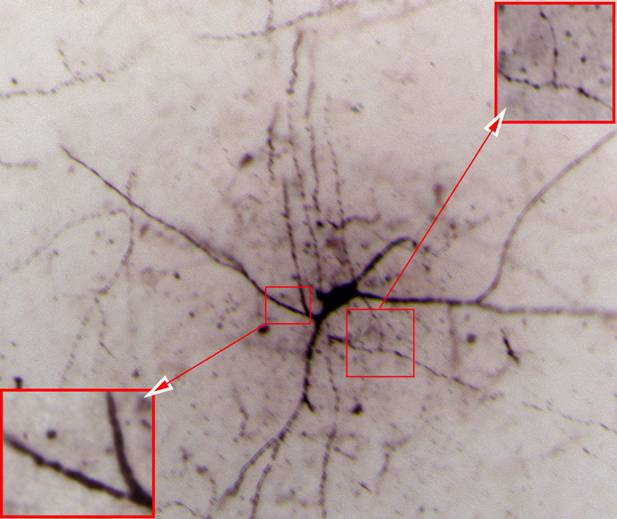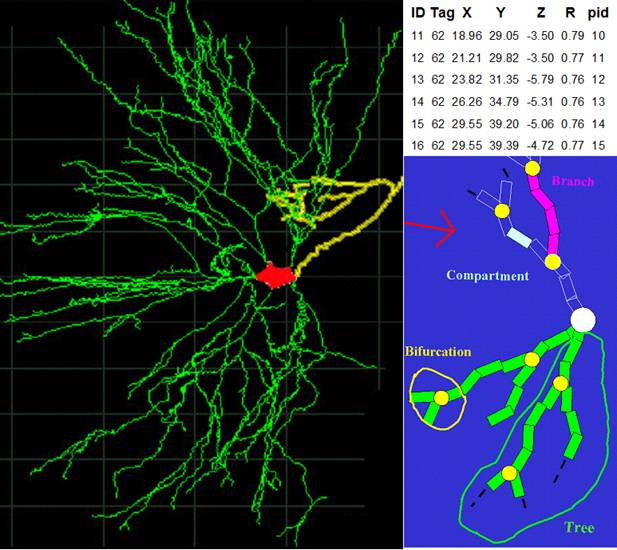### Additional Background

Each data set consists of an image stack, or list of three-dimensional voxels (X, Y, Z, intensity). An example of one such image is provided in Fig. 1, with two small areas at higher magnification highlighted in red. One location in this volume will be defined as the root (e.g. in the soma if this is visible). The digital reconstruction consists of one or more binary trees starting at the root. Each tree is represented as a set of interconnected tapering cylinders, or compartments, in a standard ASCII format (.swc). Each compartment is represented in the reconstruction file by a line of seven fields, namely a numeric identity (the line number), a service tag (to distinguish e.g. individual trees stemming from the soma), the three spatial coordinates and the radius of the cylinder's end point, and the identity of its parent compartment in the path to the root. The root is assigned a virtual parent with identity -1. Tools are publicly available to visualize, analyze, and interconvert digital reconstructions in this format. Note that this "vector" representation, which is adopted in nearly all programs for manual reconstruction from optical microscopy (e.g. Microbrightfield Neurolucida) is different from the surface rendering which results from membrane contours as commonly traced in electron microscopy.Figure 1 - Example of a raw image from a stack. Red boxes highlight zoomed-in regions.

Every compartment (except the root) has exactly one parent compartment and may have 0, 1, or 2 children compartments, corresponding respectively to a termination, continuation, or bifurcation. A node is defined as a root, bifurcation or termination in the tree. Every node is characterized by an order and a degree. The order of a node is the number of nodes in the shortest path to the root. The degree is the number of terminations a node leads to. The root has zero order, while terminations have unitary degree. For a bifurcation of order n, the two nodes of order n+1 are the children nodes, and the node of order n-1 is the parent. An example of a reconstruction (not corresponding to the previous image), a small portion of its line-by-line representation, and a schematic of these definitions is provided in Fig. 2.Figure 2 - Visualization of a digital reconstruction (left), a small portion of the corresponding file (top right) and schematic of the format representation (bottom right).